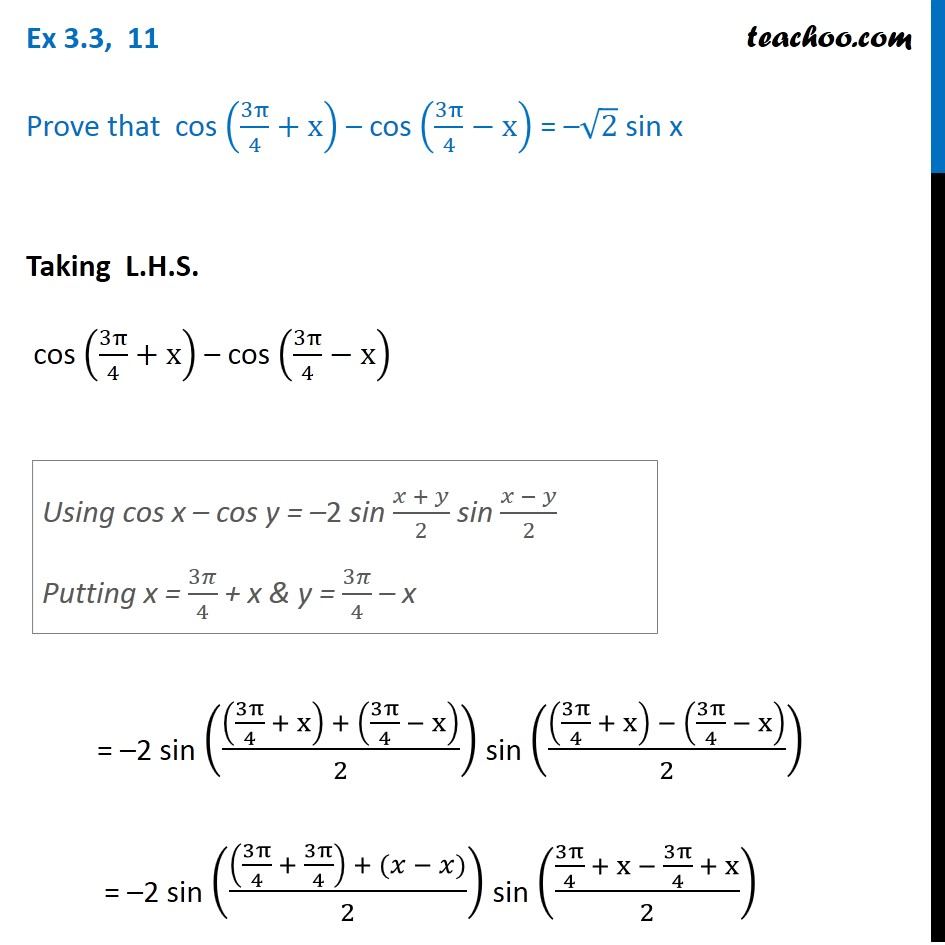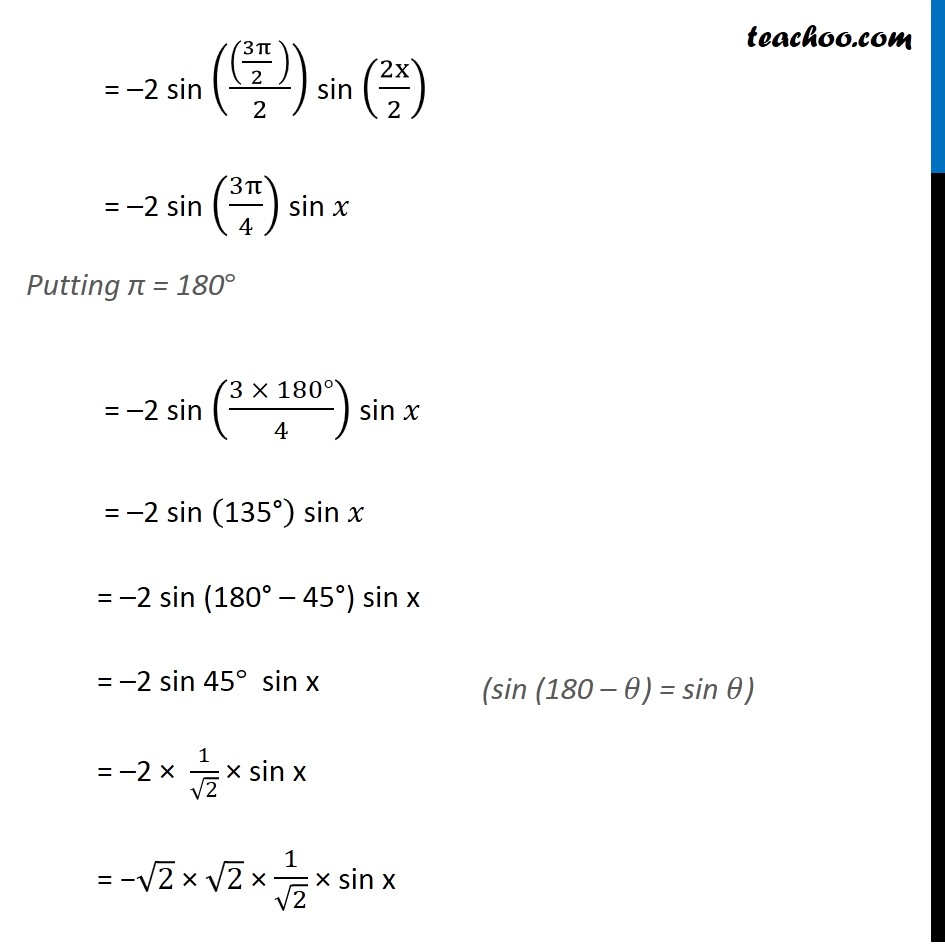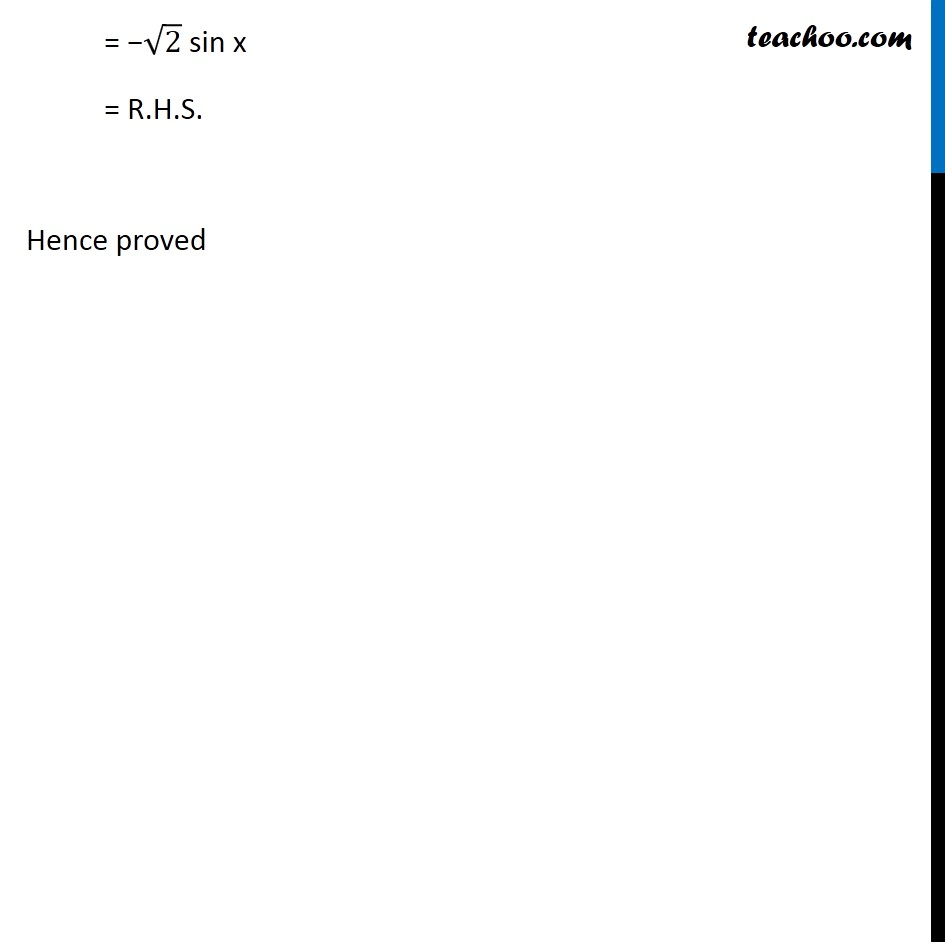Subscribe to our Youtube Channel - https://you.tube/teachoo

1. Chapter 3 Class 11 Trigonometric Functions
2. Concept wise
3. Cos x + cos y formula

Transcript

Ex 3.3, 11 Prove that cos (3π/4+x) – cos (3π/4−x) = –√2 sin x Taking L.H.S. cos (3π/4+x) – cos (3π/4−x) = –2 sin (((3π/4 + x) + (3π/4 − x))/2) sin (((3π/4 + x) − (3π/4 − x))/2) = –2 sin (((3π/4 + 3π/4) + (𝑥 − 𝑥))/2) sin ((3π/4 + x − 3π/4 + x)/2) Using cos x – cos y = –2 sin (𝑥 + 𝑦)/2 sin (𝑥 − 𝑦)/2 Putting x = 3𝜋/4 + x & y = 3𝜋/4 – x = –2 sin (((3π/2 ))/2) sin (2x/2) = –2 sin (3π/4) sin 𝑥 Putting π = 180° = –2 sin ((3 × 180°)/4) sin 𝑥 = –2 sin ("135°" ) sin 𝑥 = –2 sin (180"°" – 45"°") sin x = –2 sin 45° sin x = –2 × 1/√2 × sin x = −√2 × √2 × 1/√2 × sin x (sin (180 – 𝜃) = sin 𝜃) = −√2 sin x = R.H.S. Hence proved

Cos x + cos y formula